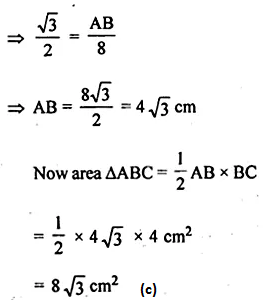# ML Aggarwal Class 10 Solutions for ICSE Maths Chapter 20 Heights and Distances MCQS

## ML Aggarwal Class 10 Solutions for ICSE Maths Chapter 20 Heights and Distances MCQS

ML Aggarwal Class 10 Solutions for ICSE Maths Chapter 20 Heights and Distances MCQS

Choose the correct answer from the given four options (1 to 9):

Question 1.
In the given figure, the length of BC is
(a) 2 √3 cm
(b) 3 √3 km
(c) 4 √3 cm
(d) 3 cmSolution: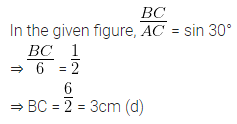Question 2.
In the given figure, if the angle of elevation is 60° and the distance AB = 10 √3 m, then the height of the tower is
(a) 20 √3 cm
(b) 10 m
(c) 30 m
(d) 30 √3 mSolution:Question 3.
If a kite is flying at a height of 40 √3 metres from the level-ground, attached to a string inclined at 60° to the horizontal, then the length of the string is
(a) 80 m
(b) 60 √3 m
(c) 80 √3 m
(d) 120 m
Solution: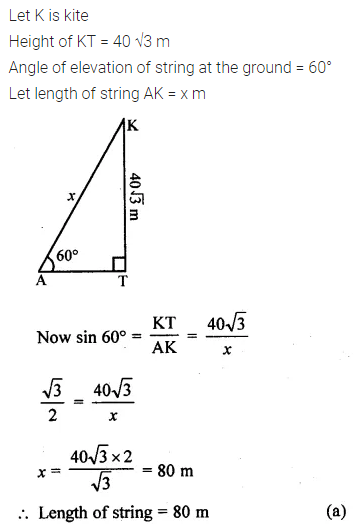Question 4.
The top of a broken tree has its top touching the ground (shown in the given figure) at a distance of 10 m from the bottom. If the angle made by the broken part with the ground is 30°, then the length of the broken part is
(a) 10 √3 m
(b) $$\frac { 20 }{ \sqrt { 3 } }$$
(c) 20 m
(d) 20 √3 mSolution:Question 5.
If the angle of depression of an object from a 75 m high tower is 30°, then the distance of the object from the tower is
(a) 25 √3 m
(b) 50√ 3 m
(c) 75 √3 m
(d) 150 m
Solution: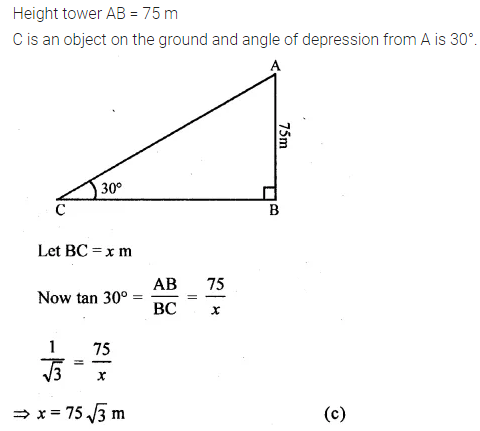Question 6.
A ladder 14 m long rests against a wall. If the foot of the ladder is 7 m from the wall, then the angle of elevation is
(a) 15°
(b) 30°
(c) 45°
(d) 60°
Solution:Question 7.
If a pole 6 m high casts shadow 2 √3 m long on the ground, then the sun’s elevation is
(a) 60°
(b) 45°
(c) 30°
(d) 90°
Solution: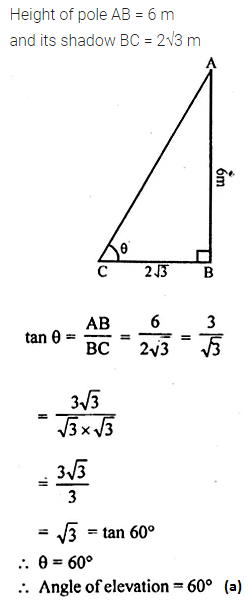Question 8.
If the length of the shadow of a tower is √3 times that of its height, then the angle of elevation of the sun is
(a) 15°
(b) 30°
(c) 45°
(d) 60°
Solution:Question 9.
In ∆ABC, ∠A = 30° and ∠B = 90°. If AC = 8 cm, then its area is
(a) 16 √3 cm²
(b) 16 m²
(c) 8 √3 cm²
(d) 6 √3 cm²
Solution: